# Mathematical formula of Vector Refraction?

Hi everyone,
The only Vector Refraction formula I could find online is:
t = √(1−μ²[1−(ni)²])n+μ[i−(ni)n]
, such that t is the refracted vector, n is the normal vector, i is the incident vector and μ = nᵢ/nₜ

Is this the formula that was implemented into Blender which is used to find the refracted vector?

• If it’s not, then what is? This is the only formula I could find online and it works pretty well too.
• If it is, please check out the following question:

I made a mathematical example here and compared it to Blender. And Blender’s Vector Refraction didn’t output the same refracted vector as the above formula:

(Figure 1)
Description:
The wall of numbers at the top is my detailed calculation of finding the refracted vector. The 2 sketches at the bottom is the comparison between my output and Blender’s output, and they are different.

• The left screenshot is a (2,2,2) cube before being applied with Vector Refraction node, the corner nearest to your eyes is i = <2,2,2> which I intended to apply the formula onto. (Also, the blue cube is the original (1,1,1) cube).
• The right screenshot is after applying n = <2,2,0> and μ = nᵢ/nₜ = 1/1.333 = 0.75 into the node. You can see that i = <2,2,2> doesn’t flip to point in the opposite direction and down, like in my expectation. It only flipped but didn’t point down.

What are your thoughts on this?
Thank you. Any help is greatly appreciated!

3 Likes

You are indeed correct! Blender’s results have been off for a year and a half and you’ve been the first person to notice. I’ll submit a patch fixing it.

5 Likes

This is my latest post which address the problem more clearly, could you please take a look?

I’m not confident with the accuracy of my calculation in this post but I’m confident with my latest post.

It’s fine, I redid your math and arrived at the same result. Not only that, but I checked the Refraction Node’s source code and it’s indeed wrong.

2 Likes

I’m told that Blender implemented OpenGL’s code as its Vector Refraction function. Here’s the code in mathematical function form: t = μ[i−(ni)n]−√(1−μ²[1−(ni)²])n

I notice that Blender’s output of refracted vector doesn’t make sense at all.

I have:

• incident vector i = <−2,−2,−2>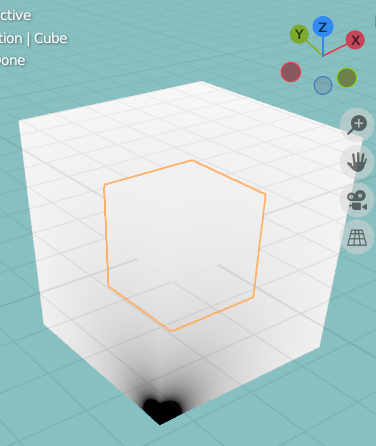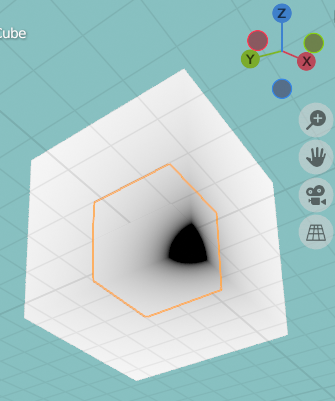the black dot you see is i = <−2,−2,−2>)

• normal vector n = <2,2,0>
• Ratio of IORs: nᵢ/nₜ = 1/1.333 = 0.75

I input those pieces of information into Vector Refraction node like this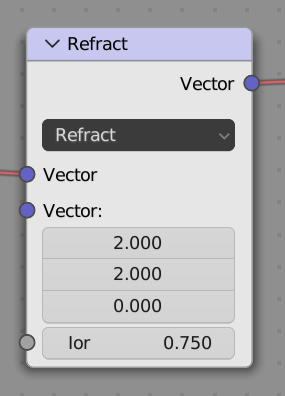So, Blender’s refracted vector looks like this: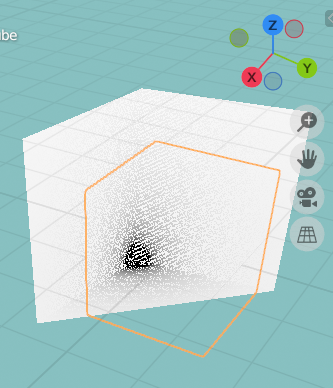, it approximately is t = <−1.5,−1.5,−1.5> which doesn’t make sense since its magnitude isn’t even equal to the incident vector’s magnitude.

My calculation, please focus on the one at the top:

The refracted vector I outputted is what I believe should be what Blender is supposed to output, not t = <−1.5,−1.5,−1.5>.

What’s wrong with Blender? Did I do something wrong?

1 Like

Moderation notice: There’s no need to have 2 different topics on this, i merged the 2.

3 Likes

It’ll be my first time contributing to Blender.
Hopefully by the end of the day the patch will be up for reviewing.

If you’re curious, what the node was doing was using the formula without first normalizing the incident vector. For the time being, you can normalize it “manually” before passing it to the Refraction Node and it should work fine.

1 Like

I would love to contribute somehow but I have no knowledge in coding.

By the way, I notice that it does normalize the normal vector, but seems like it refuse to normalize the incident vector for some reason.
Hopefully it will normalize both vectors after receiving your patch.

1 Like

Getting all set up and building blender from source took longer than I had anticipated!

Not only that, but I’d like to take the time to make the vector refraction formula more consistent on the edge cases: when the normal vector is 0, when the angle of the normal and the incident vector is larger than pi/2, etc. I might open a “design task” to see what the developers think the intended behaviour should be.

That’s all to say, the issue that was bothering you has been dealt with (currently on my computer, only), but the overall fix might take a little longer to land.

1 Like

And for the sake of precision, I’d like to note that, while the formulas you’ve used on both posts are correct (the first one assumes the normal and the incident vectors have the same “overall direction”: the normal “points downwards” from the surface, thus (n · i) >= 0; the second one assumes the opposite, the normal “pointing upwards” from the medium interface), the usual convention in physics is the one you’ve used in the second image: if the normal vector is (2,2,0), the incident vector must be coming “from above”, (-2,-2,-2).

Since — as far as I can see, given the second convention — the formula is meaningless if the normal and incident vector have the same “overall direction” (as the incident ray would be coming from inside the surface it wants to enter), it might be a good idea to think of the normal vector as an “undirected vector”, and adjust its sign during the calculations to always make sure that (n · i) <= 0. This would prevent users from making meaningless calculations, and from having to guess which convention is used with regards to the direction of the normal. On the other hand, this behaviour might be slightly unexpected, and some users may find the “impossible angles” useful (?) for some arcane stuff.

What do you think? I’ll ask the blender devs as well.

1 Like

Good quality things sure take longer and more effort to make. You should do whatever you feel necessary since you understand the problem way better than me. In the mean time, I can just apply Vector Normalization before applying Vector Refraction, and then scale the vectors back up again.

1 Like

Hi! I just want to update that after normalizing the incident vector, the node outputs correct refracted vector now.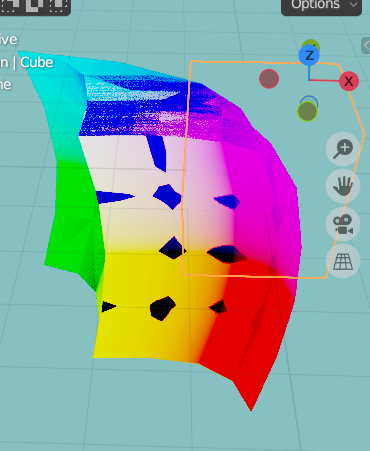The black dot is approximately at t = <−2.206,−2.206,−1.496>, which is exactly equal to my output in my calculation:

Here’s my node setup (sorry for light theme):

1 Like

Thank you for the explanation!
Sorry for late reply. I was at school.

What do you think?

Sorry, I don’t think I can provide anything useful as I’m inferior in these subjects compared to you. I’m currently talking to a Physicist, I will tell you anything that I find useful after talking to him.

So I have to 2 formulas:
t = (n(ni)−i)nᵢ/nₜ−n√(1−(1−(ni)²)(nᵢ/nₜ)²)
t = (in(ni))nᵢ/nₜ−n√(1−(1−(ni)²)(nᵢ/nₜ)²)

I don’t think I’m gonna use first one since it’s not OpenGL’s formula (which is what I’m told to be what Blender implemented as Vector Refraction), even though it outputs correct refracted vector. And the second one is what I transcribed from OpenGL’s code so I’m gonna use it.

Another reason I’m avoiding it is because its direction of the incident vector is not theoretically correct (as you said, in Physics, the incident vector is supposed to point inwards, but here, its incident vector is derived to point outwards).

Also, its in and tn has different signs (whereas my latest calculation uses OpenGL’s formula which outputs same sign for both in and tn. Why should I care? Because tn should always have the same sign as in, as refraction doesn’t change the direction (whereas for reflection, nr should always have the opposite sign to in).

Here are the derivations of the first formula, hope you’ll find them useful, you can see that in all the diagrams of these derivations, i is derived to point outwards:

1. Slidetodoc.com (Derivation appears after clicking “next” 35 times)
2. Ohio state edu (At slide 12)
3. Stack Exchange (Images in the replies - it’s from a book)

I can’t find any derivation of the second one (OpenGL), apparently no one has ever derived it.

the formula is meaningless if the normal and incident vector have the same “overall direction”

Does it mean that my second calculation here doesn’t serve any purpose? Does it mean that, as long as ni ≤ 0 , the function has a chance to output correct refracted vector and ni > 0 will output incorrect vector?

I notice that, the side of the box whose incident vectors have opposite “overall direction” to the normal vector get refracted and outputted like that. But the side of the box whose incident vectors have the same “overall direction” to the normal vector just disappears. Actually those vectors don’t disappear but are overlaying the refracted vector, like this:What do you think?

I’ll ask the blender devs as well

Yes sir. Do as you please.

2 Likes

Glad to know the workaround is working nicely (sort of)!

Things get a bit confusing when we’re trying to talk in terms of (n · i), etc., as it all depends on the convention for the normal vector we’ve adopted. We can sidestep the confusion by talking directly about what matters: the angle the light rays make with the normal direction (see Θ_1 and Θ_2 in the figure attached to my previous comment.)

The formula is meaningless if the normal and incident vector have the same “overall direction”

Translating to angles: The formula is meaningless if Θ_1 > 90,
and it’s not hard to see why: the light ray would not be able to cross the interface in the “right direction” coming at that angle. The current node does return a value in such cases nonetheless, and it’s entirely up to the user making sure the inputs make sense.

1 Like

Should you like to follow the development process first hand,
I’ve submitted a formal bug report here: ⚓ T102068 Vector Math's "Refract" node gives wrong results.
And have proposed a solution here: ⚙ D16341 Fix T102068: Refract node gave wrong results

2 Likes

I don’t know what the process of contributing to Blender is like, but if your submission got accepted by the developers, it would be great. If not, you have already done a good job, man. Everyone appreciates your efforts.

I talked to a user on Reddit, they think that not normalizing the incident vector was intentional for the purpose of making the refraction process less computational expensive. This is their opinion on this situation:
“… it takes computational energy to normalize a vector, to the tune of at least a square-root each time, so if the function does not need a vector to be normalized to do it’s job, or it can have normalized vectors as a precondition of the inputs you feed to it, then it’s up to you to decide where and when normalization needs to be done. The only “bug” I see here is at most a failure to document and clearly advertise which inputs must be normalized.”

Still, I don’t understand why Blender automatically normalized normal vector but not incident vector, it is just as computationally costly.

1 Like

That’s true in that I was a bit hasty in characterizing it as a bug.

We’ve copied the function from OpenGL, and OpenGL indeed is so extremely focused on performance that it skips all the safety checks, and delegates the task of making sure the calculations make sense to the user. That’s fine, as the intended users of OpenGL are technical people, who may want performance and fine-grained control over their calculations above everything else.

Blender and Geometry Nodes, however, are made for artists and non-technical people. As such, we’re reasonably expected to deliver much more polished, user-friendly tools. In this context, providing a node which returns meaningless results for the majority of inputs seems absolutely unacceptable to me. Normalizing a single input vector isn’t terribly computationally expensive, anyway.

2 Likes

The current node does return a value in such cases nonetheless

Yeah I just calculated the situation where θ₁ of Snell’s Law > 90°, and the formula actually does output a refracted vector, and Blender’s result is the same as the formula’s output, but the refracted vector’s direction makes it look nothing like a refraction.

This is my calculation and Blender’s displacement: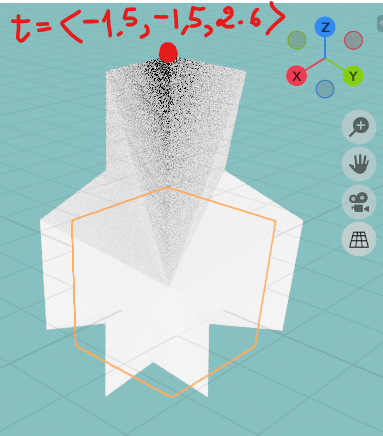One question: Does Total Internal Reflection exist in Blender?
I’m told that, in order for Total Internal Reflection to occur, the Square root of OpenGL’s formula
t = μ[i−(ni)n]− √(1−μ²[1−(ni)²]) n
has to be negative, hence there’ll be Imaginary number.
But if that’s true, how does the formula even output a (Real valued) vector in that situation since there’s an Imaginary number inside it?
Does Blender even work with Imaginary number?

When a light ray hits a transparent medium such as glass, it splits into two: a bit of it enters the glass (the refracted ray), and a bit of it bounces off the glass (the reflected ray).
It’s always true that the energy of the original ray is conserved, that is:

intensity_of_incident_ray = (intensity_of_refracted_ray) + (intensity_of_reflected_ray),

but this energy need not be evenly split between the two new rays: it depends entirely on the angle the incident ray hits the glass (our old Θ_1). So, for instance, if the incidence angle is 0°, most of the light will be refracted, while the reflected ray will be barely visible. And as the incidence angle increases, the reflected ray gets stronger, while the refracted one gets dimmer.

If the incoming ray is travelling from a higher-IOR to a lower-IOR material (such as glass to air), there’s even an incidence angle past which the refracted ray ceases to exist, and all the incoming light gets reflected: that’s the point of total internal reflection (internal because we’re “inside” the glass, try to go “out” to open air).

What does that have to do with our formula? Well, it’s true that the formula we have only gives us information about the direction of the refracted ray, not about its intensity. But the incidence angle in which the refracted intensity becomes zero is exactly the same one which causes the refracted angle to be 90°. Once we’re past that point there’s no more refracted ray, and our formula breaks. That’s why you’re getting imaginary answers.

It seems like you were expecting the formula to give you the reflected ray in that case. It won’t: our formula is for the direction of the refracted ray, only. There’s a different formula for the direction of the reflected ray (a very simple one), and two other ones (four, actually) for their respective intensities.
The ratio of reflected to refracted (or “transmitted”) light is given by the “Fresnel coefficients”, should you like to look them up. But then things gets a bit technical rather quickly.

Try playing with this applet to get a feel for the phenomena (if you’re trying to find the point of total reflection, remember you should go from a high-IOR medium to a low-IOR one. It’ll be clear why when you see it).

1 Like

It’s great you’re curious about stuff, but this is a forum meant for blender development topics. If you have more questions, we can chat about them in a more informal place (blender.community, blender.chat)

1 Like